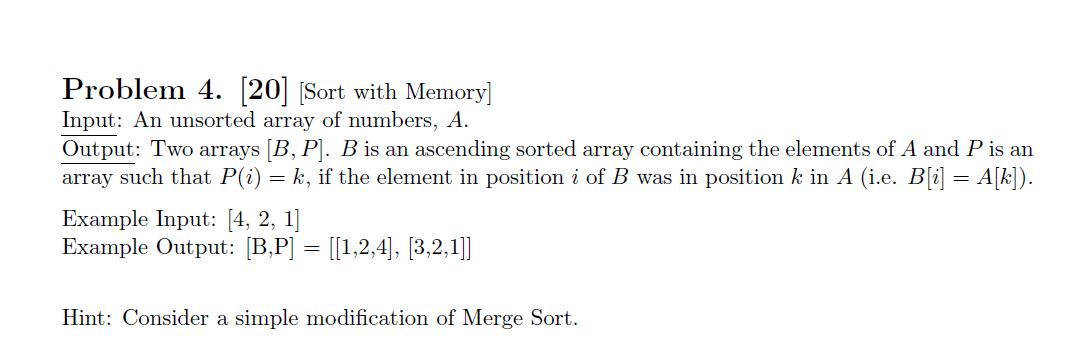# Problem 4. 20 Sort with Memory] Input: An unsorted array of numbers, A Output: Two arrays [B, P. B is an ascending sorted array containing the elements of A and P is an array such that P(i) k, if the element in position i of B was in position k in A (i.e. B[i] A[k]) = Example Input: [4, 2, 1] Example Output: B,P [1,2,4], [3,2,1]] Hint: Consider a simple modification of Merge Sort

Questionhelp_outlineImage TranscriptioncloseProblem 4. 20 Sort with Memory] Input: An unsorted array of numbers, A Output: Two arrays [B, P. B is an ascending sorted array containing the elements of A and P is an array such that P(i) k, if the element in position i of B was in position k in A (i.e. B[i] A[k]) = Example Input: [4, 2, 1] Example Output: B,P [1,2,4], [3,2,1]] Hint: Consider a simple modification of Merge Sort fullscreen

### Want to see this answer and more?

Experts are waiting 24/7 to provide step-by-step solutions in as fast as 30 minutes!*

*Response times vary by subject and question complexity. Median response time is 34 minutes and may be longer for new subjects.
Tagged in
EngineeringComputer Engineering

### Programing